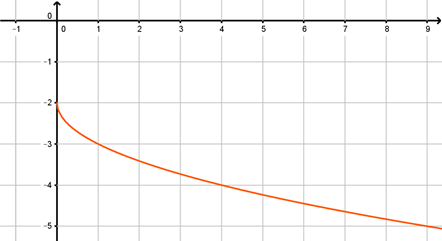• The learning process is entertaining and exciting
• The solved tasks turn into coordinates
• Along with your schoolmates and friends, go on an adventure, searching for hideouts
• E-mail:

# 2718.2. Function y=√x

#### Write the displayed functions formula!y=\sqrt{x}

8
Subject
Algebra
Theme
Square roots
Subtheme
Function y=√x

#### Solve tasks to find hidden Geo point coordinates

1. Algebra: Fraction addition and subtraction.
2. Chemistry: Waters chemical properties and formation.
3. Physics: Movement diagram.
4. Algebra: Square root
5. Physics: Lighting and safety.
6. Physics: Light spectre and colour formation.
7. Algebra: Transformation of algebraic expressions.
8. Chemistry: Substance oxidation process and reactions.
9. Algebra: Square root
10. Algebra: Square trinomial roots